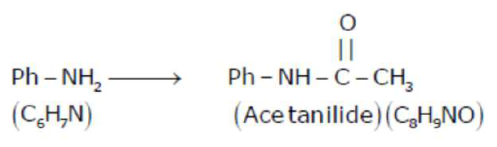# 1.86 g of aniline completely reacts to form acetanilide.

Question:

$1.86 \mathrm{~g}$ of aniline completely reacts to form acetanilide. $10 \%$ of the product is lost during purificatiion. Amount of acetanilide obtained after purification (in g) is _____________$\times 10^{-2}$

Solution:

(243)Molar mass $=93$ Molar mass $=135$

$93 \mathrm{~g}$ Aniline produce $135 \mathrm{~g}$ acetanilide

$1.86 \mathrm{~g}$ produce $\frac{135 \times 1.86}{93}=2.70 \mathrm{~g}$

At $10 \%$ loss, $90 \%$ product will be formed after purification.

Amount of product obtained $=\frac{2.70 \times 90}{100}=2.43 \mathrm{~g}=243 \times 10^{-2} \mathrm{~g}$